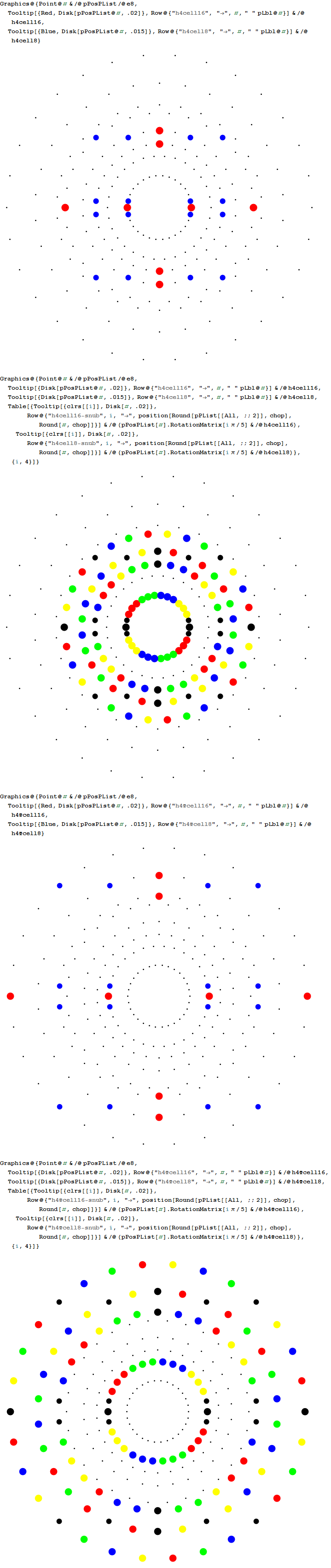# 3D Printed E7 1_32 Polytope Projected to the E6/F4 Coxeter Plane

The 3D model of the polytope: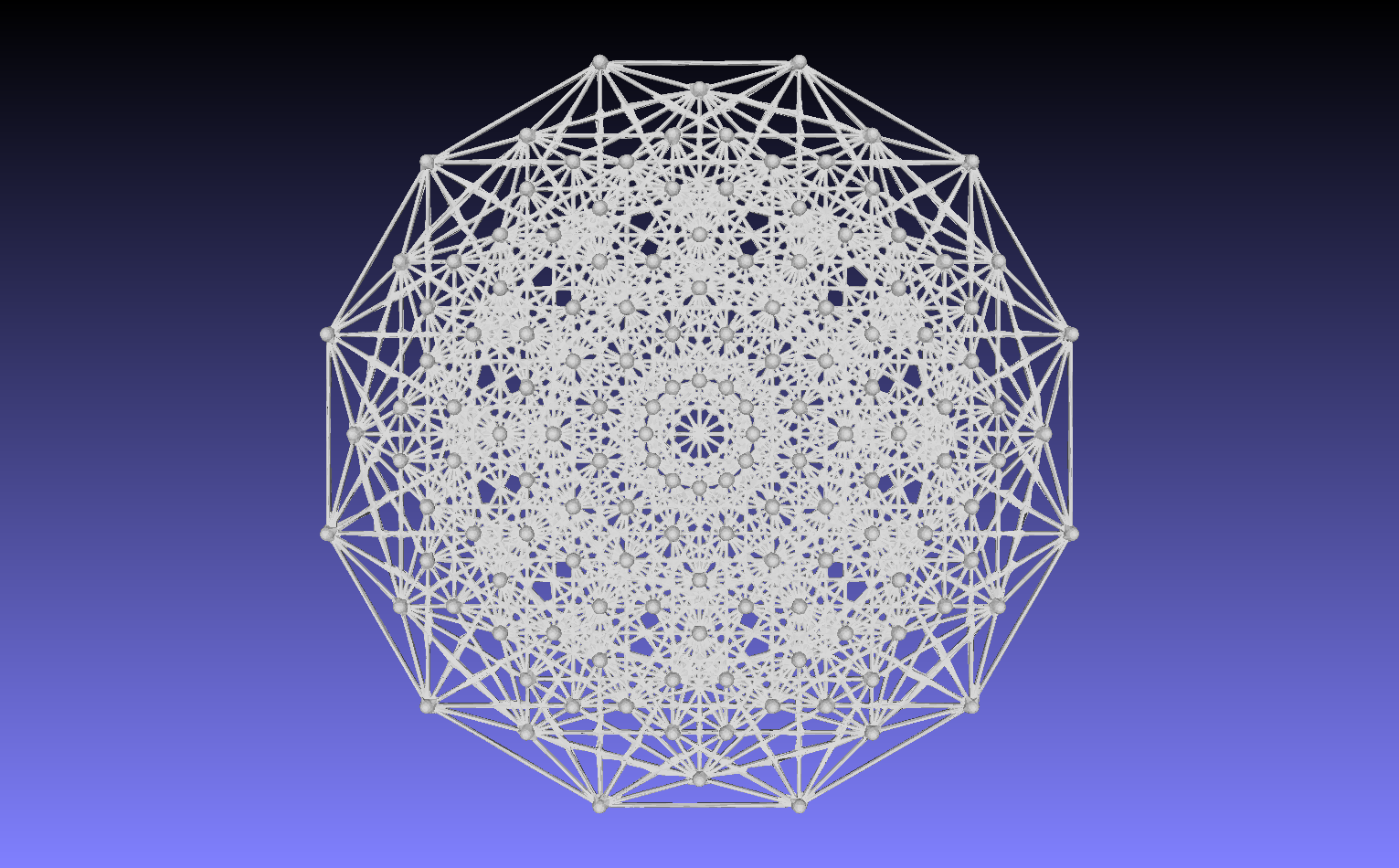3D printed in raw bronze:
Front: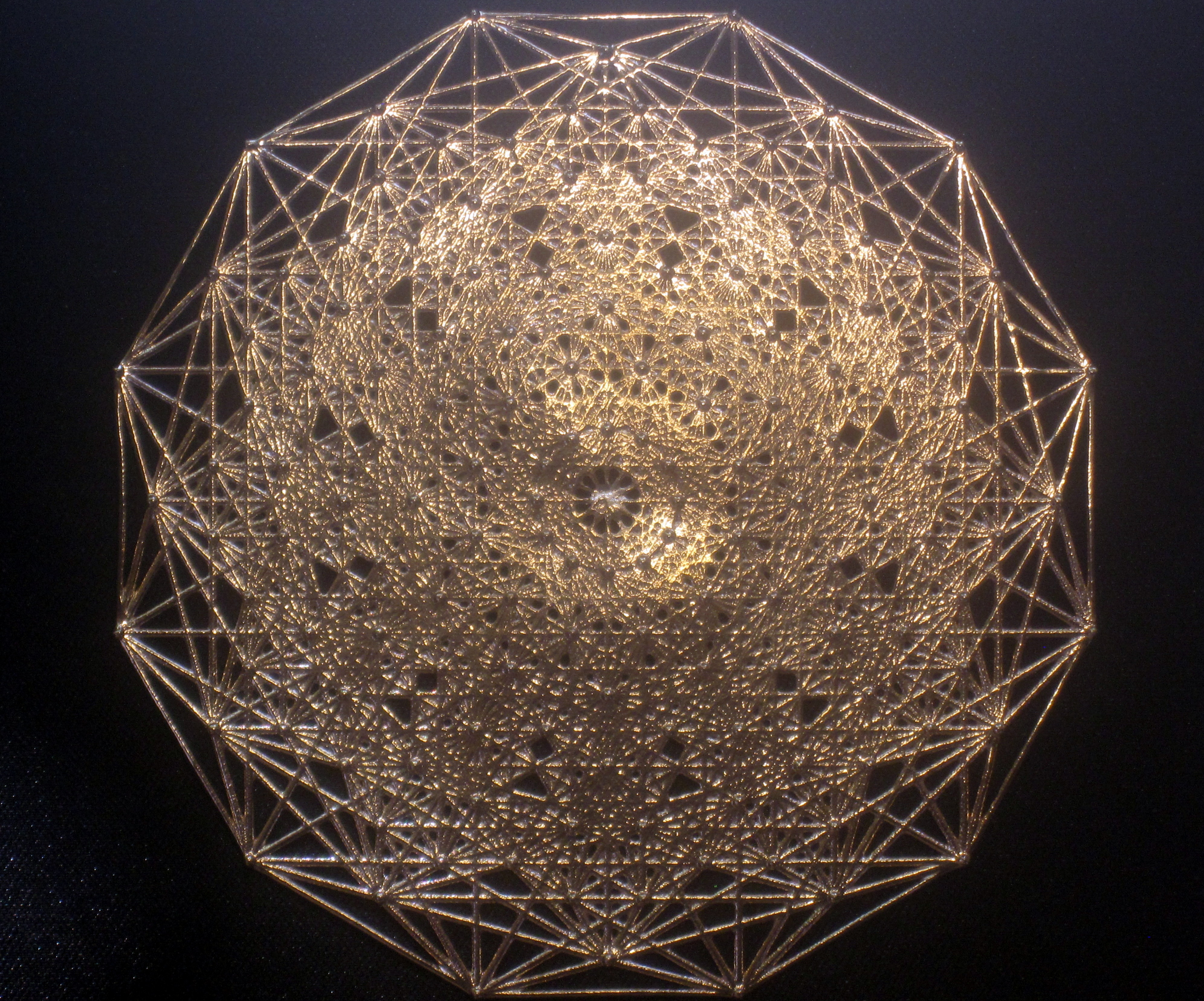Back:Here it is in a 3 1/8″x3 1/8″ laser etched optical crystal.# G2, F4, E6, E7, and E8 triality relations in 3D concentric hulls of E8

Here are the G2 gluons connected by their trialities shown in a 3D concentric hull projection of E8 using the E8 to H4 folding matrix basis vectors. This is the 4th hull, which is the outer hull of the inner H4 600 cell (an icosadodecahedron). For more on E8 hulls, see this post.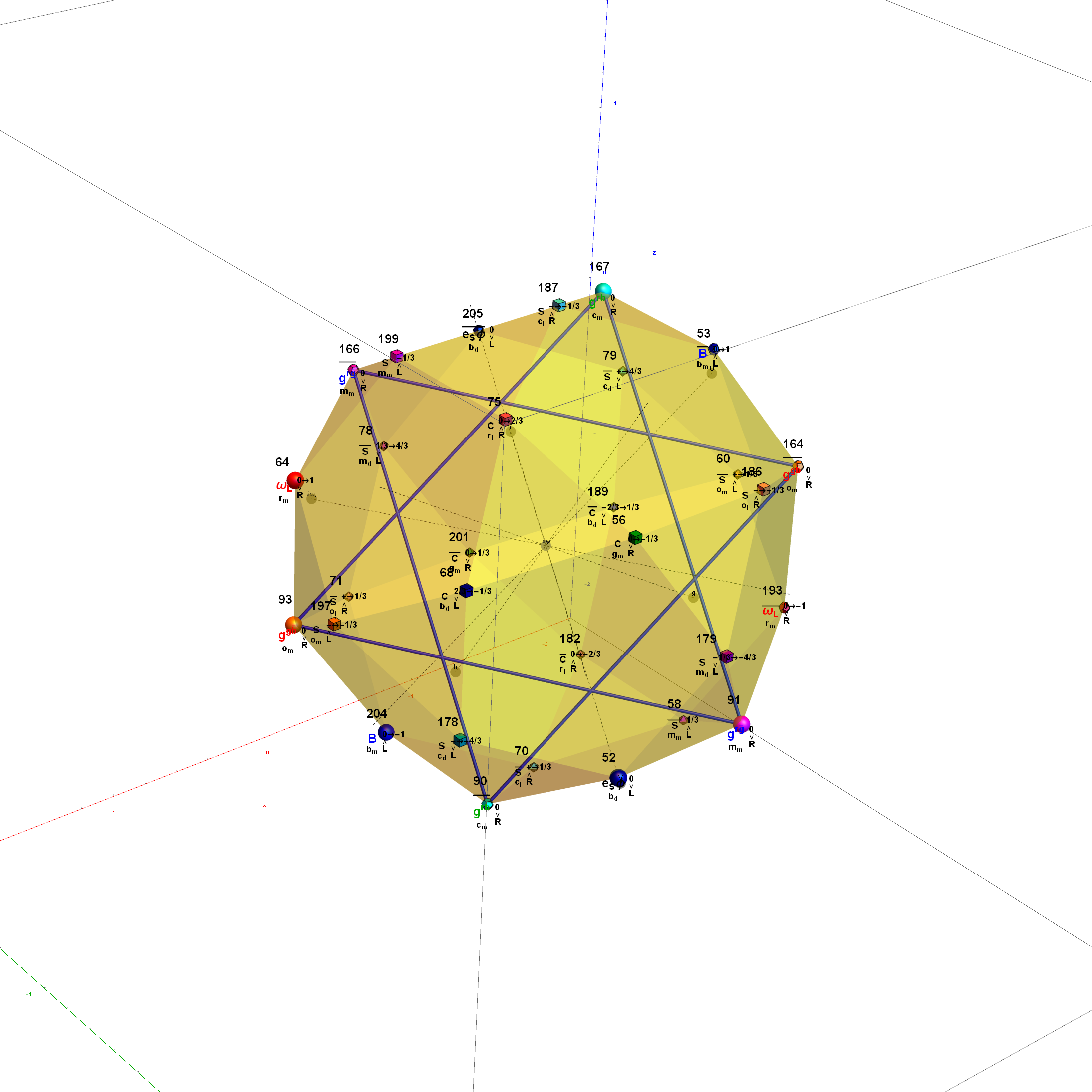The full F4 group with 10 T2 and 12 T4 trialities affecting the bosons is contained in the outer icosadodecahedron (1st hull) combined with the 3rd (quad icosahedral) and 4th icosadodecahedron hulls.

It is interesting to note that the two icosadodecahedron hulls comprise the 60 vertices of D6.Below is the full E8 with all trialities shown in 3D concentric hull projection.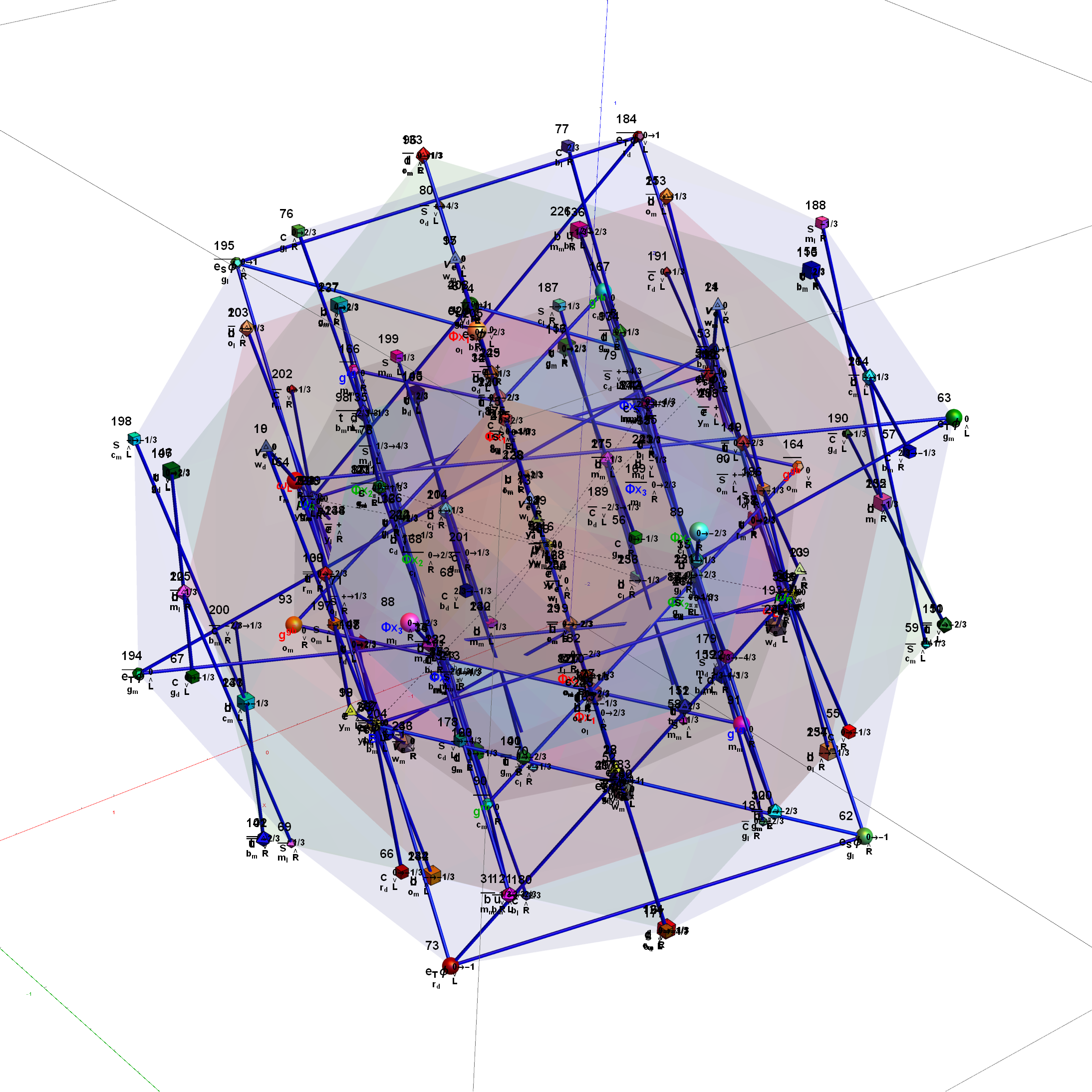#### Wow – that is nice! Remember, you heard it here first!

BTW – if you find this information useful, or provide any portion of it to others, PLEASE make sure you cite this post. If you feel a blog post citation would not be an acceptable form for academic research papers, I would be glad to clean it up and put it into LaTex format in order to provide it to arXiv (with your academic sponsorship) or Vixra. Just send me a note at: jgmoxness@theoryofeverthing.org.

# Star of David Projection Basis for E8, E7 and E6

For a full discussion of the particle assignment symmetries involved, see http://vixra.org/pdf/1503.0190v1.pdf

E8 as 240 split real even vertices with  vertex color, size, shape, and labels from a modified A.G. Lisi particle assignment algorithm. The vertex number is the position in the Pascal Triangle (Clifford Algebra) representation of E8. The blue lines forming equilateral triangles represent the  10 Bosonic (or Color) Triality (T2) and 76 Fermionic (T4) rotations (using the 8×8 matrices shown below) that transform each member of the triangle into the next member.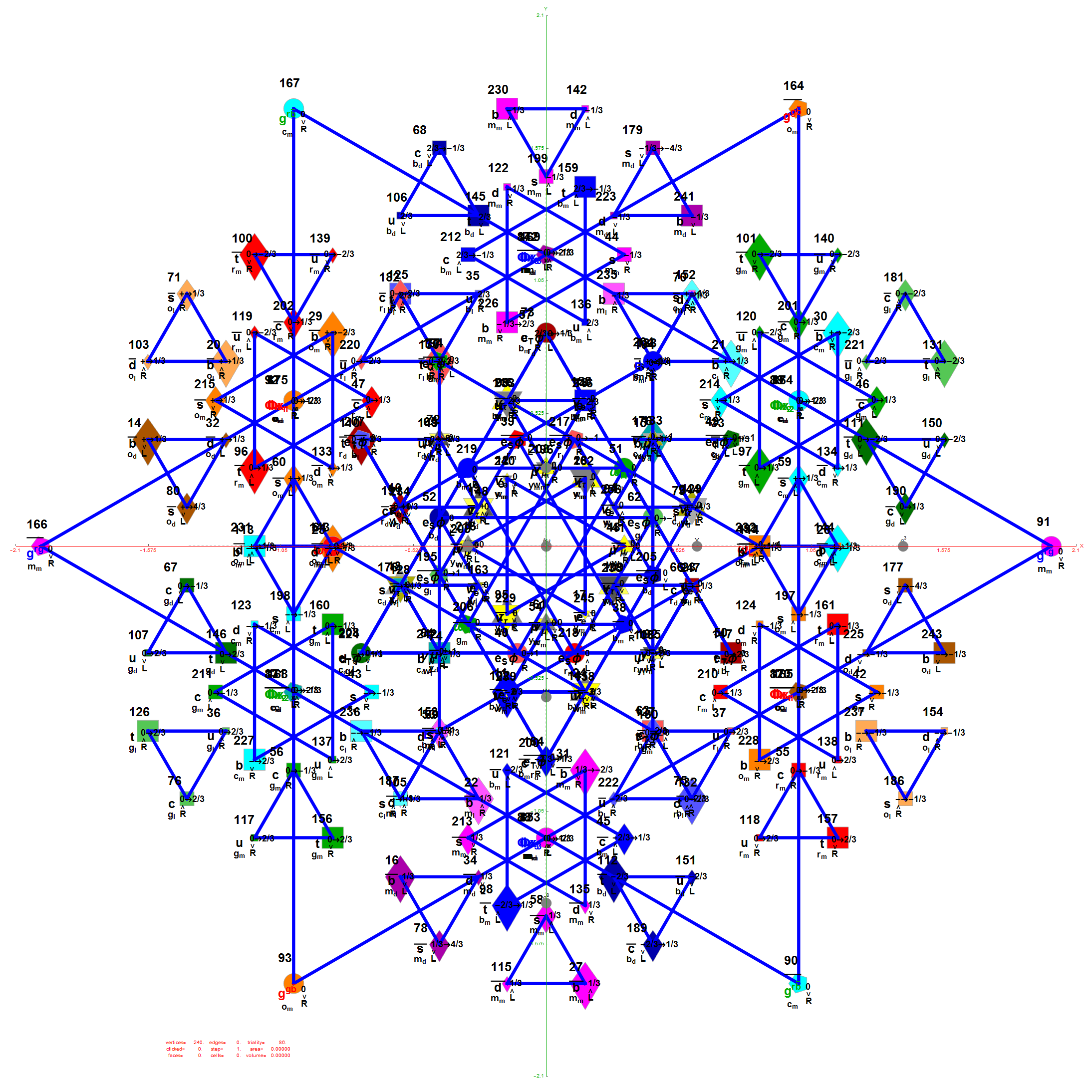E7 as derived from E8 above by taking the vertices of E8 with the last two columns being equal.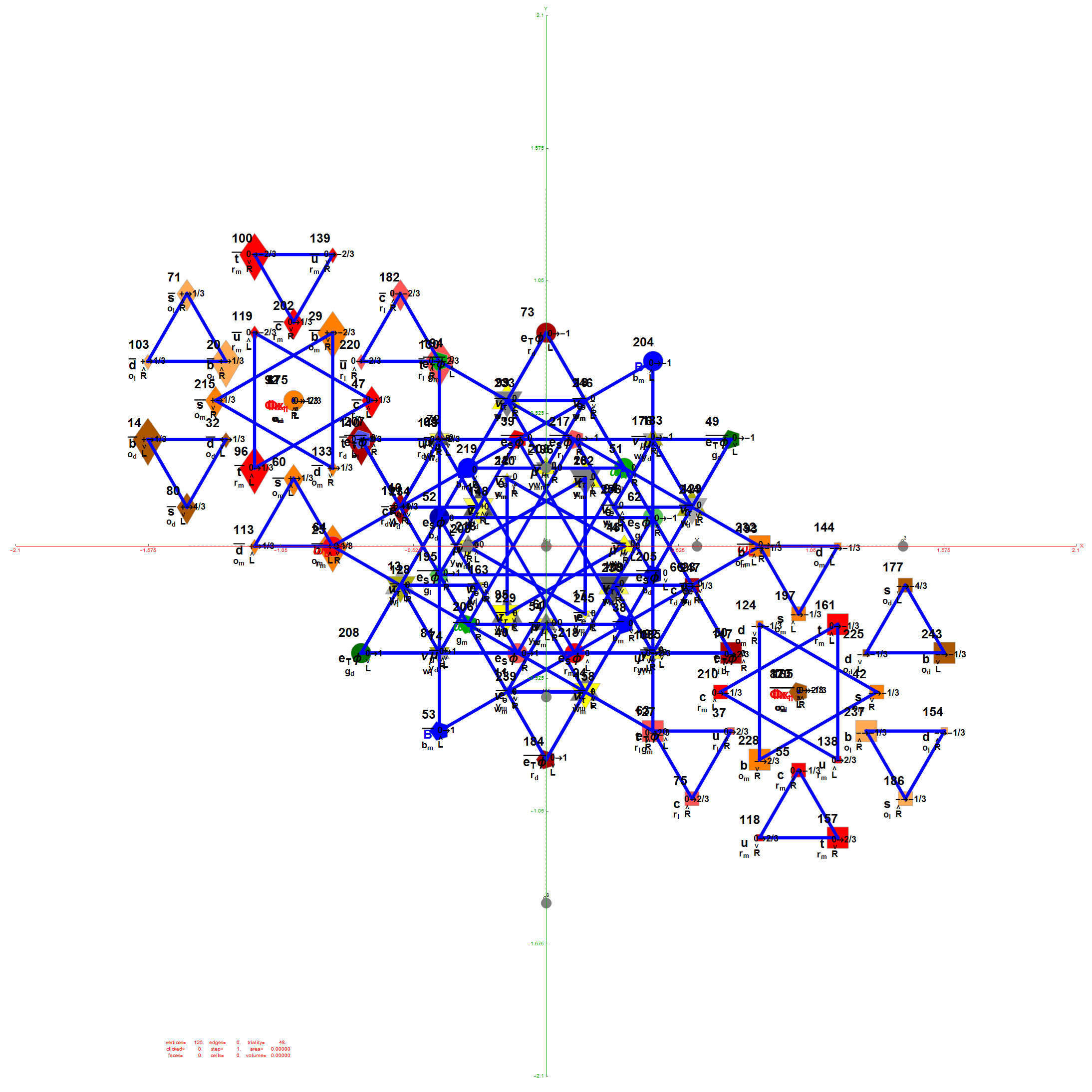E6 as derived from E8 above by taking the vertices of E8 with the last three columns being equal.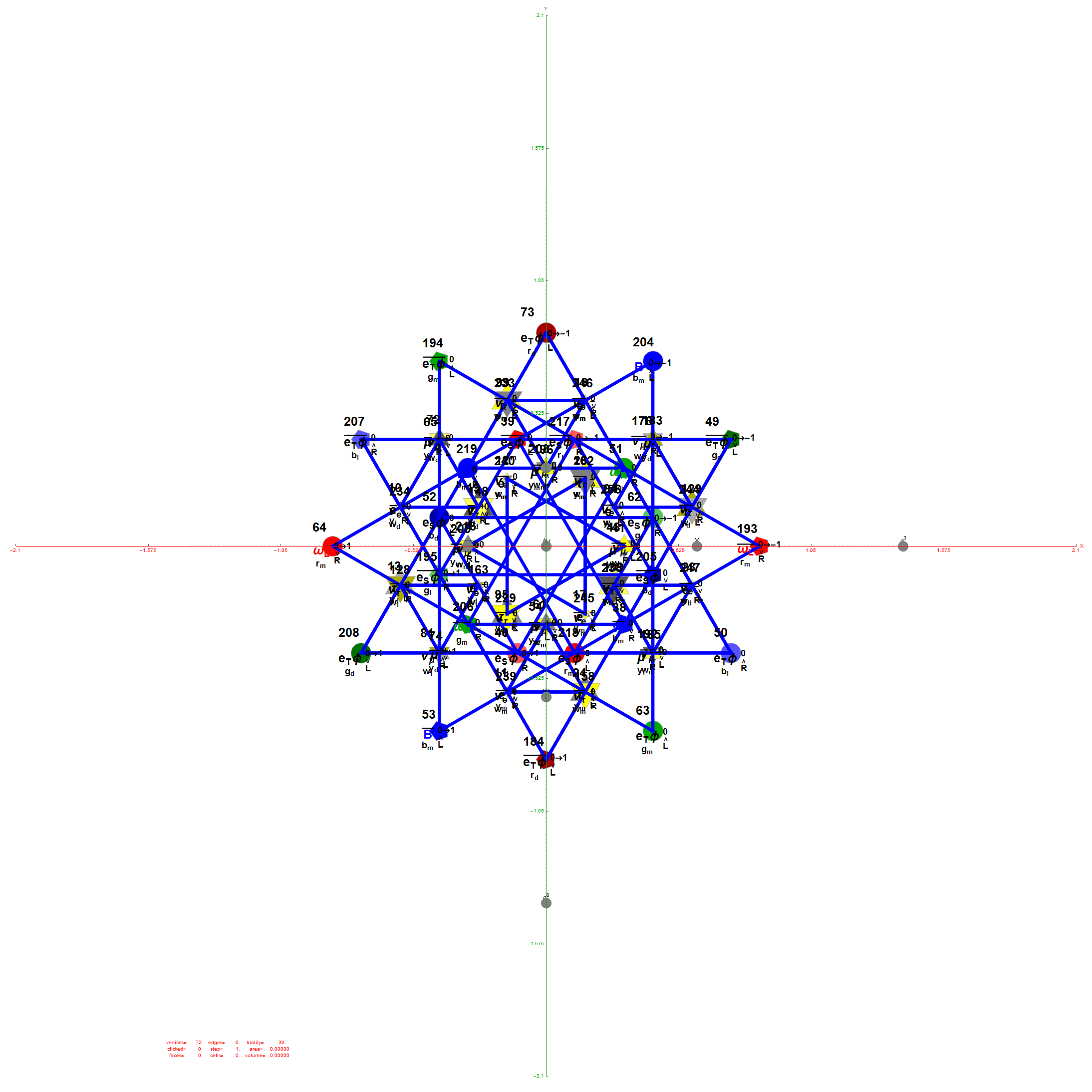The 48 vertices of F4 are made up of the 112 (D8) +/-1 integer pair vertices that are assigned to bosonic particles, which includes 22 trialities (10 from T2 and 12 from T4). The other 64 of 112  D8  vertices are assigned to the 2nd generation of Fermions. F4 is shown below with its sub-group G2 assigned to the gluons in the larger triality triangles.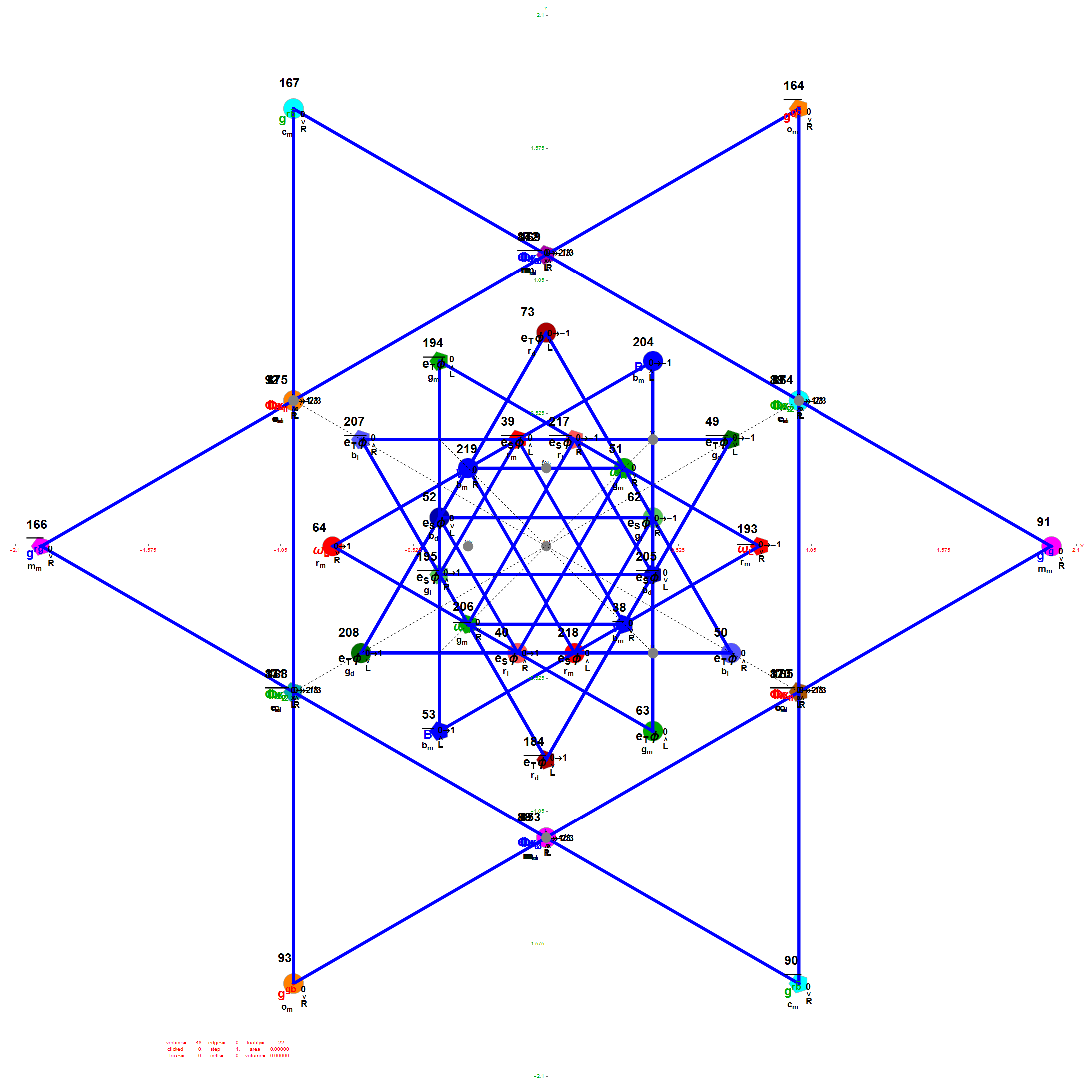Projection Basis for X and Y are H (first row) and V (second row) in the matrix below respectively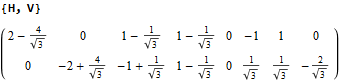The Triality Matrices are: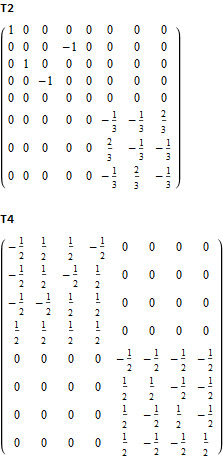The 10 Bosonic (Color) Triality Rotation Matrix (T2) equilateral triangles exclusively affect the particles that are derived from F4.

T2 rotates through the bosonic colors indicated by the last 3 columns of the E8 vertices appropriately labeled (r,g,b).The 76 Fermionic (Generation) Triality Rotation Matrix (T4) equilateral triangles. There are 64 fermion particle trialities that are derived from the 128 (BC8) half integer vertex assignments plus the 64 fermions assigned from the 112 (D8) +/-1 integer pair vertices. There is always one integer (2nd Generation) and two 1/2 integer vertices (1st and 3rd Generation) in every fermionic triality.

That is, T4 rotates through the fermionic generations.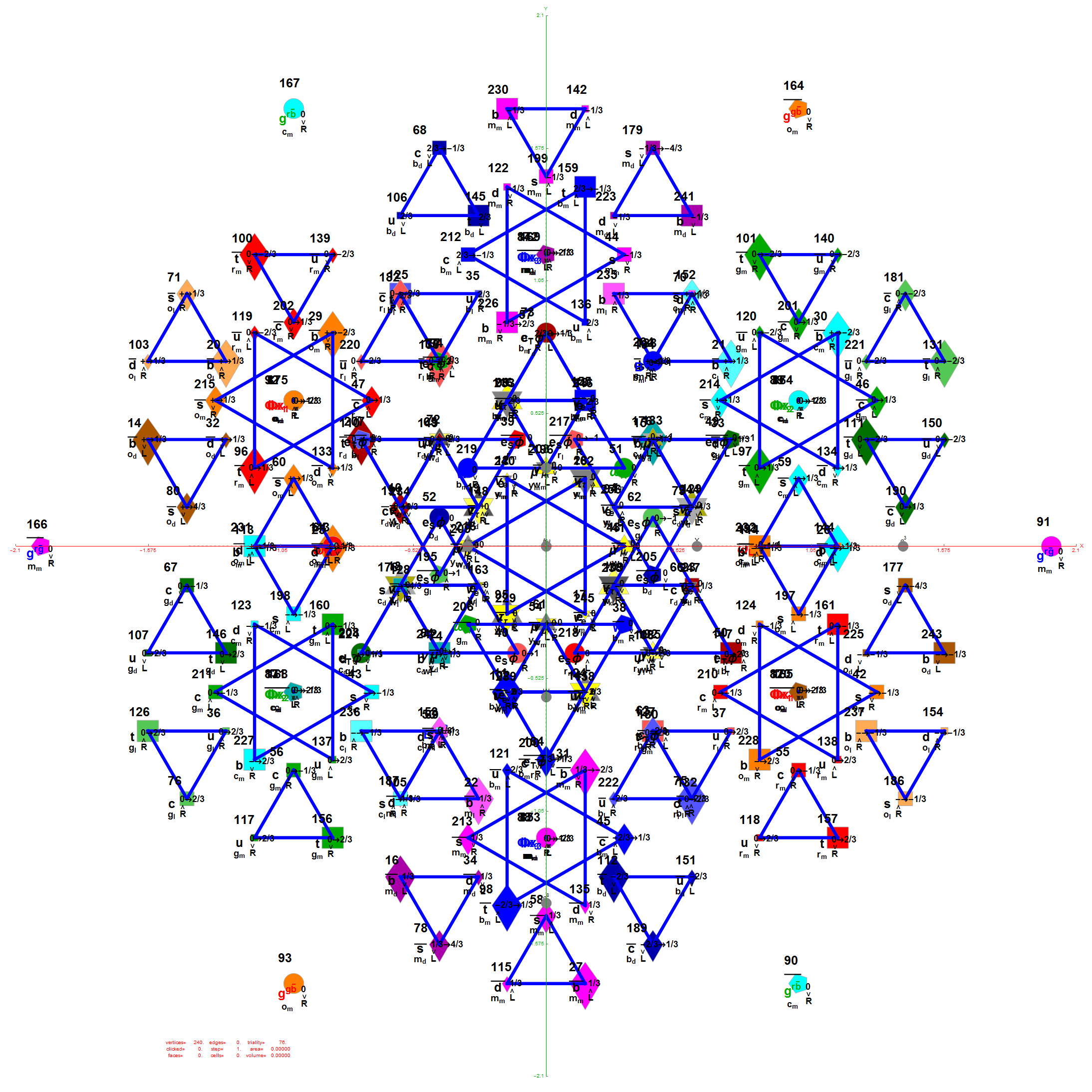There are 12 trialities from these 76 that are associated with the inner E6 & F4 Lie Group vertices assigned to bosons, shown below with only the 112 (D8) +/-1 integer pair unlabeled vertices: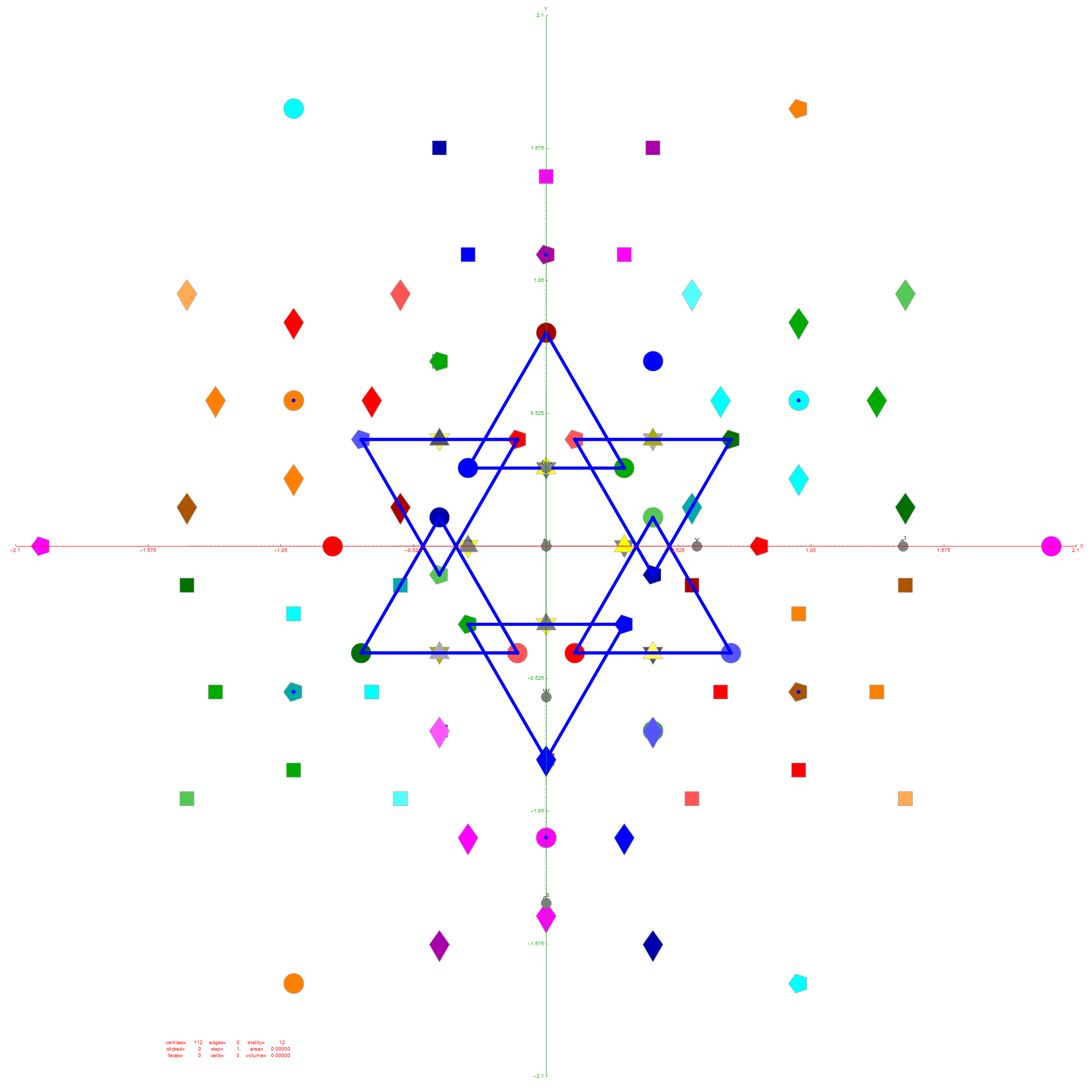Of course, there are a number of overlapping vertices in this projection stemming from the 112 (D8) +/-1 Bosonic sector. The following image colors the vertices by overlap count, with 120 Yellow with no overlaps, 24 Cyan with 2 overlaps (48), and 24 Cyan with 3 overlaps (72).

Notice the 8 projection basis vectors with dark gray circles. Of course, these projection basis vectors are the 8 “generator vertices” of E8 vertices with permutations of {+1,0,0,0,0,0,0,0} giving it the full 240+8=248 dimension count. Add to that the -1 “anti-generators” being the opposite end of the projection basis vectors and you get the full 256 vertices representing the full Clifford Algebra on the 9th row of the Pascal Triangle.There are 6720 edges in E8 with an 8D length Sqrt.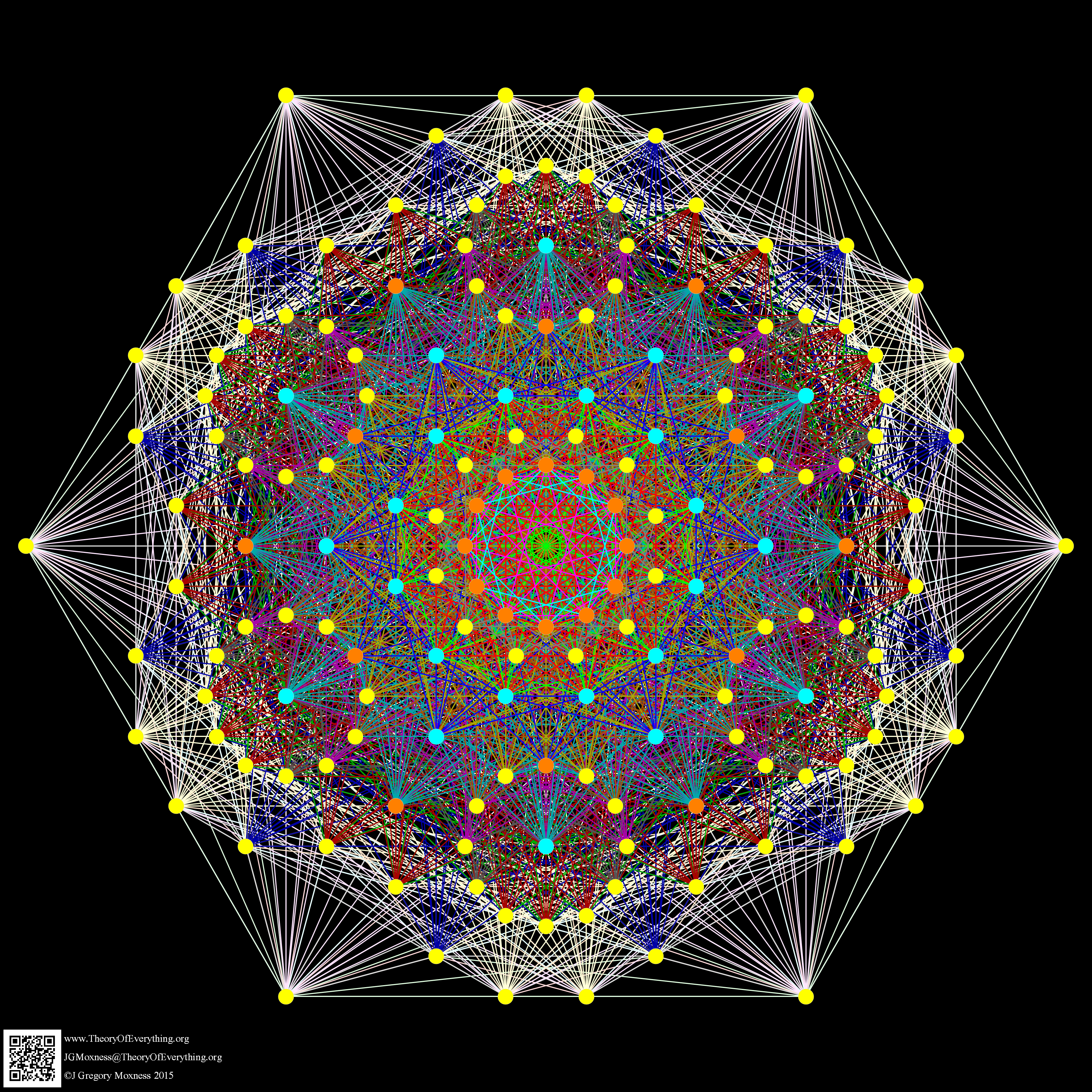Just for fun, I introduce to you the 6720 rectified E8 vertices taken from the midpoint of each edge and using the same projection with coloring of the spheres based on overlap count. Enjoy!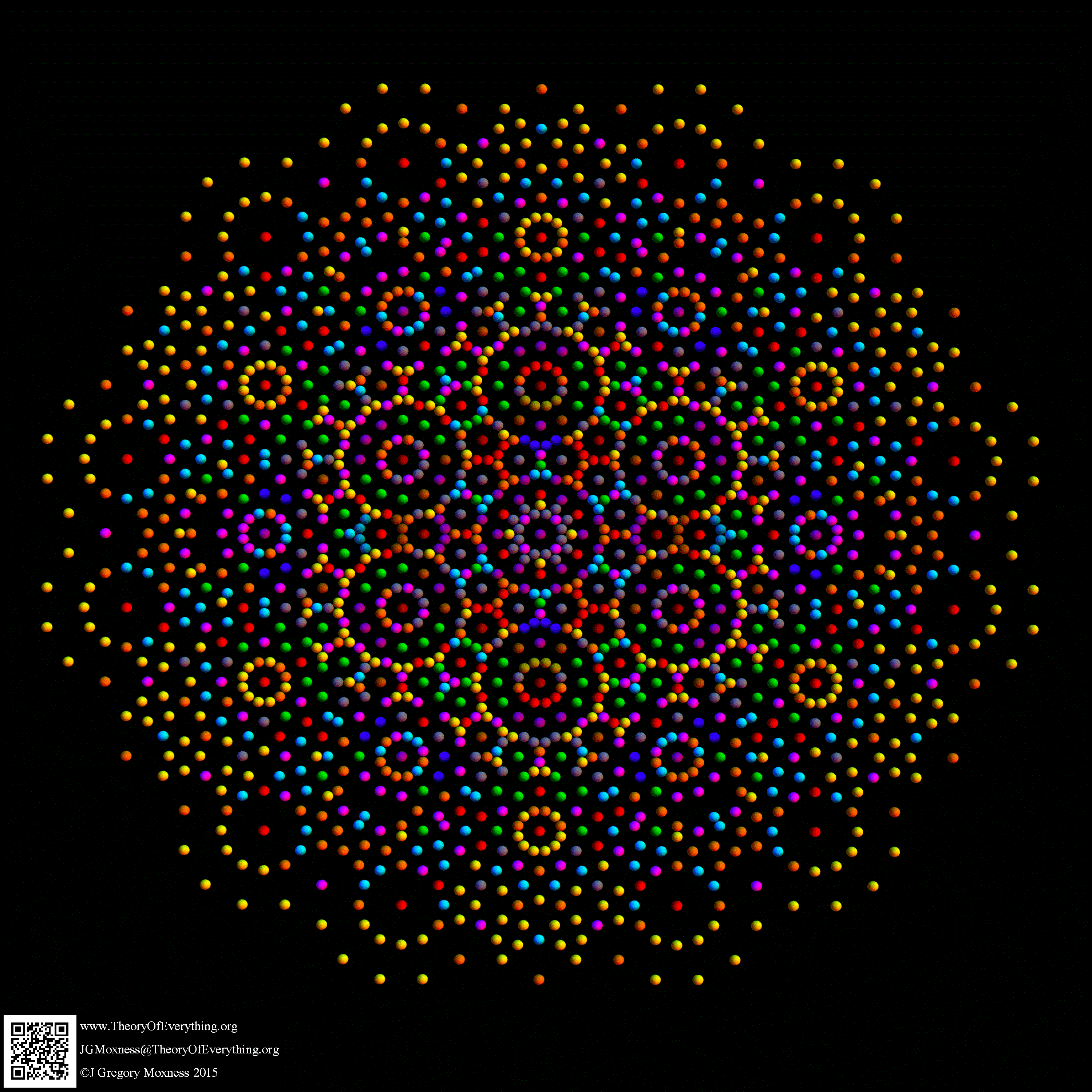Here are the G2 gluons connected by their trialities shown in a 3D concentric hull projection of E8 using the E8 to H4 folding matrix basis vectors. This is the 4th hull, which is the outer hull of the inner H4 600 cell (an icosadodecahedron). For more on E8 hulls, see this post.The full F4 group with 10 T2 and 12 T4 trialities affecting the bosons is contained in the outer icosadodecahedron (1st hull) combined with the 3rd (quad icosahedral) and 4th icosadodecahedron hulls.Below is the full E8 with all trialities shown in 3D concentric hull projection.#### Wow – that is nice! Remember, you heard it here first!

BTW – if you find this information useful, or provide any portion of it to others, PLEASE make sure you cite this post. If you feel a blog post citation would not be an acceptable form for academic research papers, I would be glad to clean it up and put it into LaTex format in order to provide it to arXiv (with your academic sponsorship) or Vixra. Just send me a note at:  jgmoxness@theoryofeverthing.org.

# The Rhombic Triacontahedron and E8 to H4+H4Φ folding

I was asked to clarify a particular projection of the Rhombic Triacontahedron from the 6-Cube subgroup of E8, which had I created for WikiPedia (WP) back in 2011 based on an E8 to H4+H4Φ rotation matrix I discovered in 2010.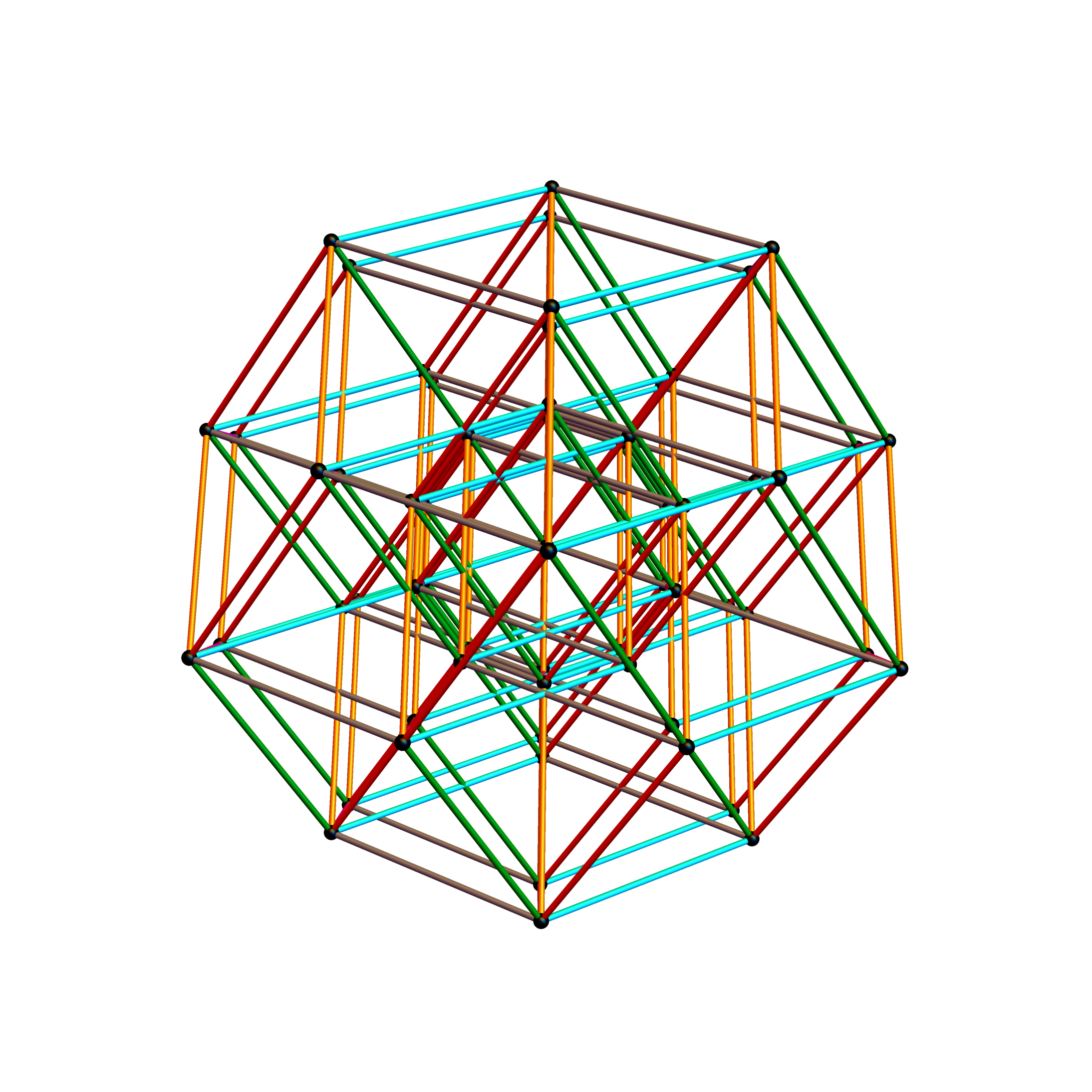The edge coloring on the projection above is defined by which of the 6 dimensional axis the edge aligns with.

BTW – if you find this information useful, or provide any portion of it to others, PLEASE make sure you cite this post. If you feel a blog post citation would not be an acceptable form for academic research papers, I would be glad to clean it up and put it into LaTex format in order to provide it to arXiv (with your academic sponsorship) or Vixra. Just send me a note at:  jgmoxness@theoryofeverthing.org.

The following projection (basis) vectors take the 6-Cube as a subset of E8 and projects it into 3D using the following basis vectors: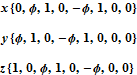Notice the last two dimensions in each vector are 0, which effectively takes the 128 8D 1/2 integer vertices of the E8 BC8 DemiCube into the 6D 6-Cube.

For the following data analysis, it is useful to reference the full list of E8 and H4+H4Φ data at the end of my blog post here.

#### The clarification desired was due to a conjecture that since E8 folds to H4+H4Φ (600 cells) and D6 folds to H3+H3Φ, that the 6-Cube should fold to an outer and inner rhombic triacontahedron scaled by Φ.

Below is a visualization of D6 folding into 2 concentric H3 icosadodecahedrons at a ratio of Φ using the same projection as described above. The edging displays the  480 Norm’d 6D Sqrt length edges, clearly showing the outer and inner H3 polytopes.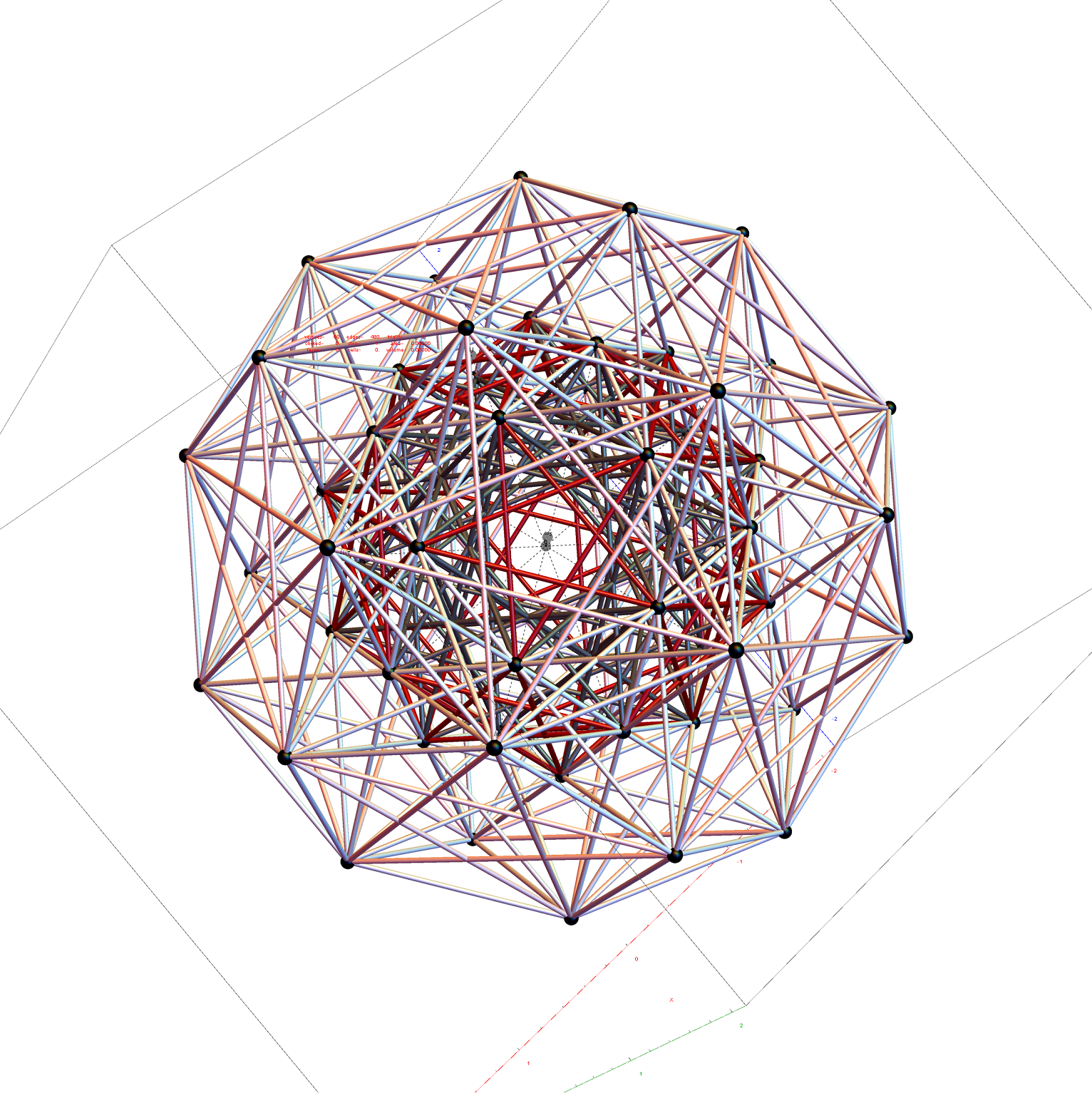Shown with exterior faces on edges (not a pure convex hull algorithm)…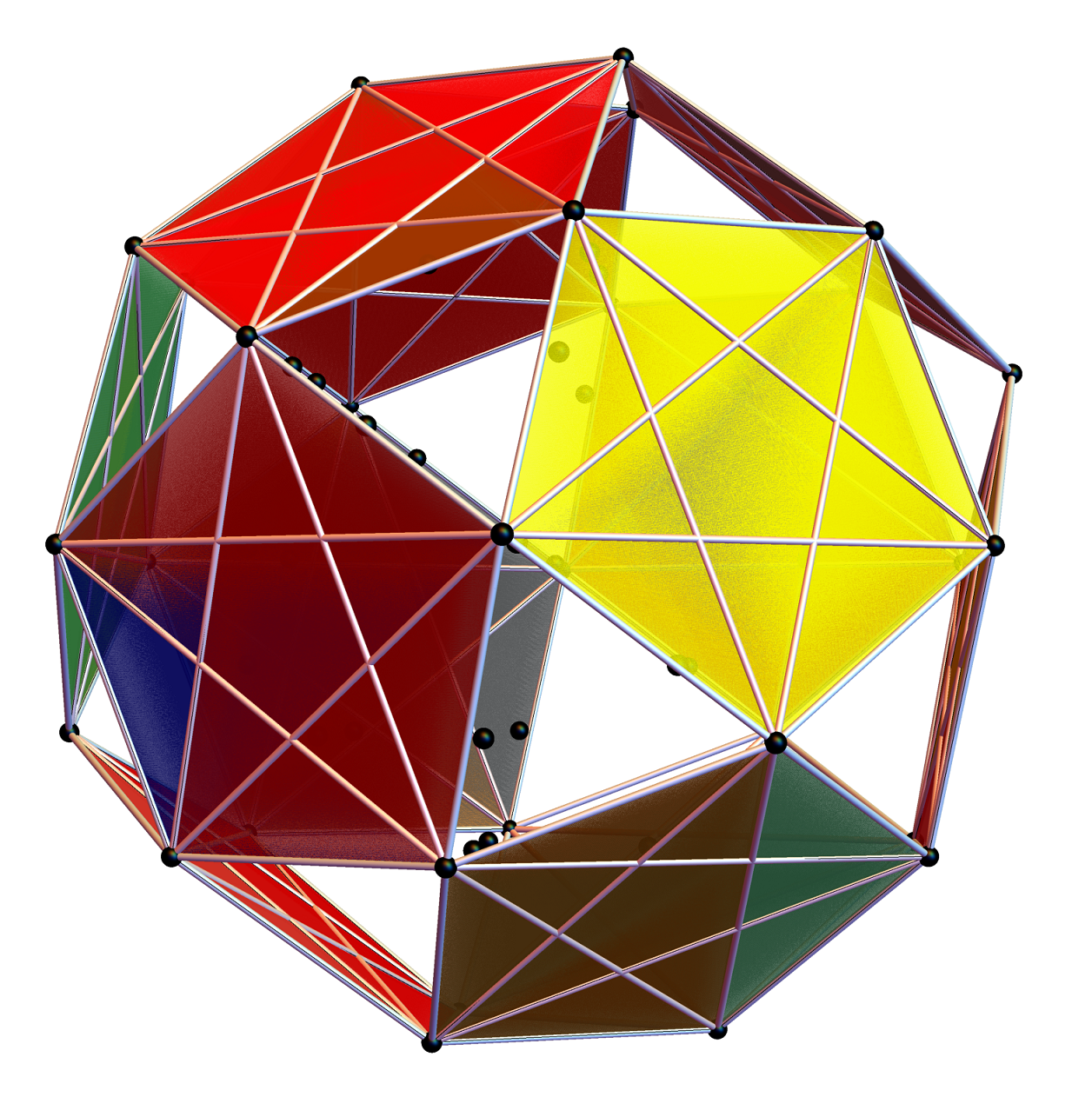The data below shows two sets of 30 vertices (outer on the left and inner on the right). They are sorted by the 3D Norm’d length of the vertex ray from {0,0,0}, followed by the vertex number in the E8 to H4+H4Φ list at the end of the post referenced above, along with the 6D E8 vertex that is a member of the D6 set, and the {x,y,z} projected vertex positionThe last line of the data set shows that the first set of outer 30 projected vertex locations on the left is numerically equal to the second inner set after multiplication by Φ.#### I did the same for the Rhombic Triacontahedron projected out of the 6-Cube.

I separated the outer and inner vertices used to make the WP projection at the top of the post. There are 64 vertices and 192 6D unit length edges forming pentagonal symmetry along specific axis (as well as hexagonal symmetries on other axis). It is clear that scaling the inner 32 vertex locations by Φ is not going to give the outer 32 vertex positions. Yet there is a similarity- they both have 12 vertices of one 3D Norm’d length and 20 of another.

Outer 32 vertices and 60 unit length Norm’d 6D edges.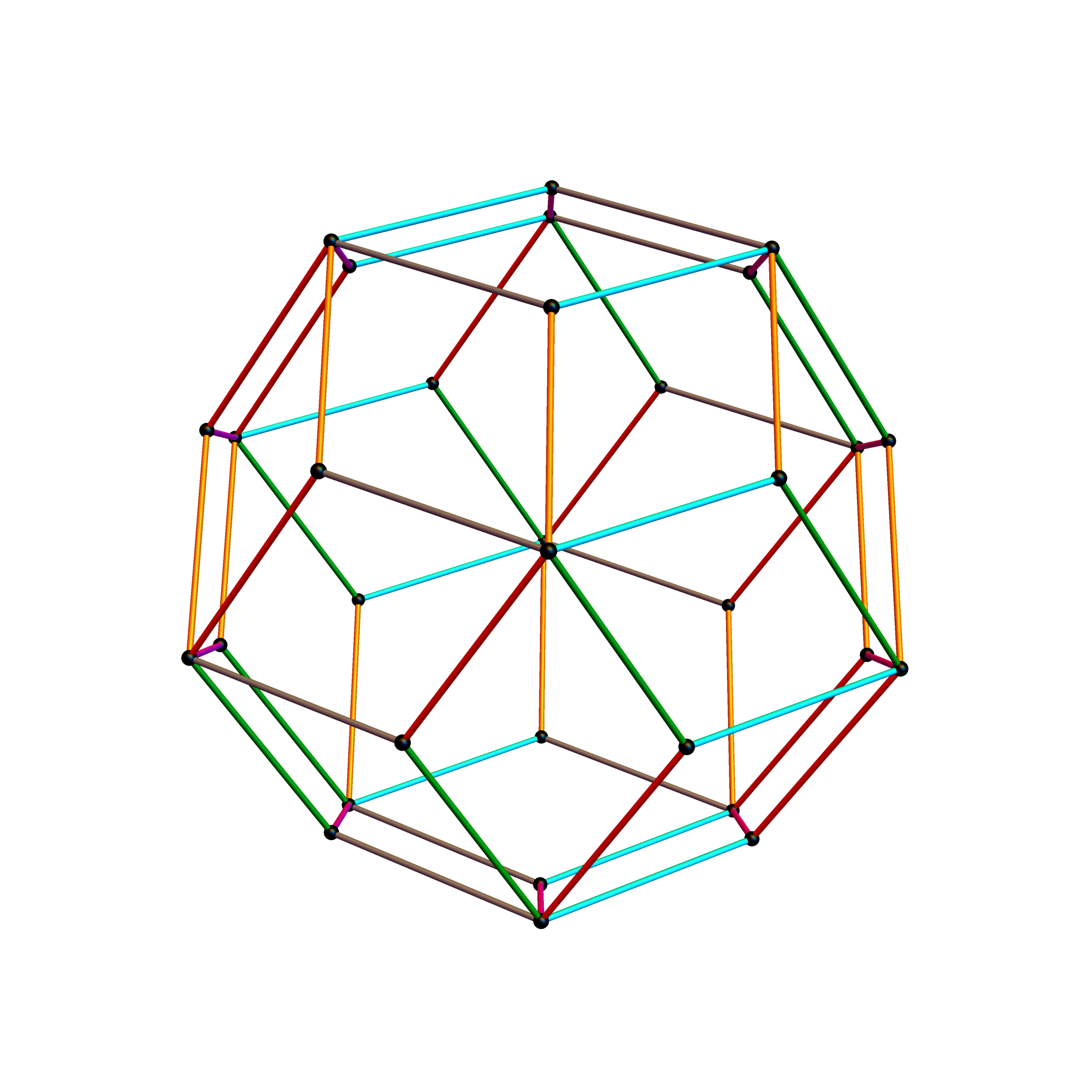The edge coloring on the projection above is defined by which of the 6 dimensional axis the edge aligns with.

Inner 32 vertices and 60 unit length Norm’d 6D edges.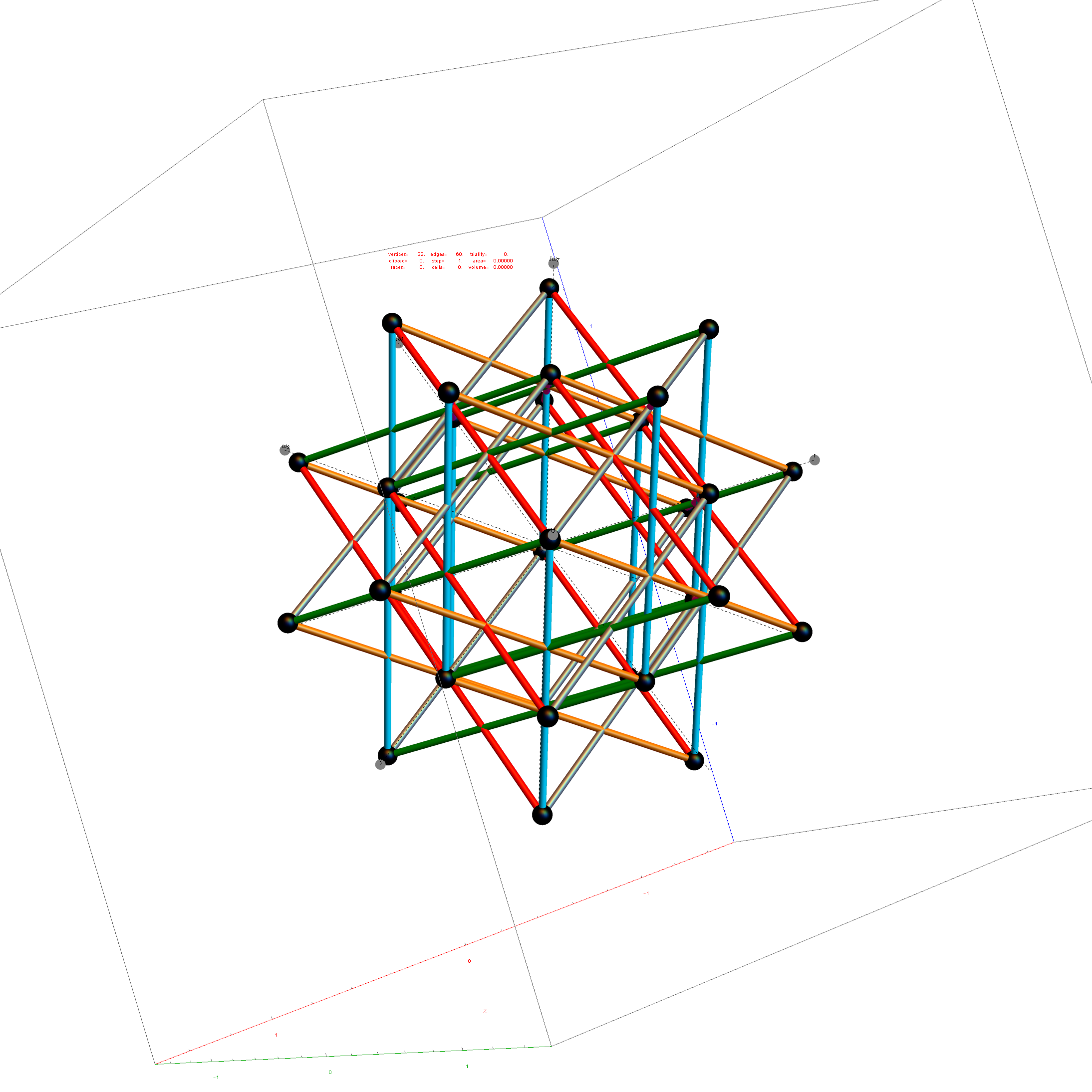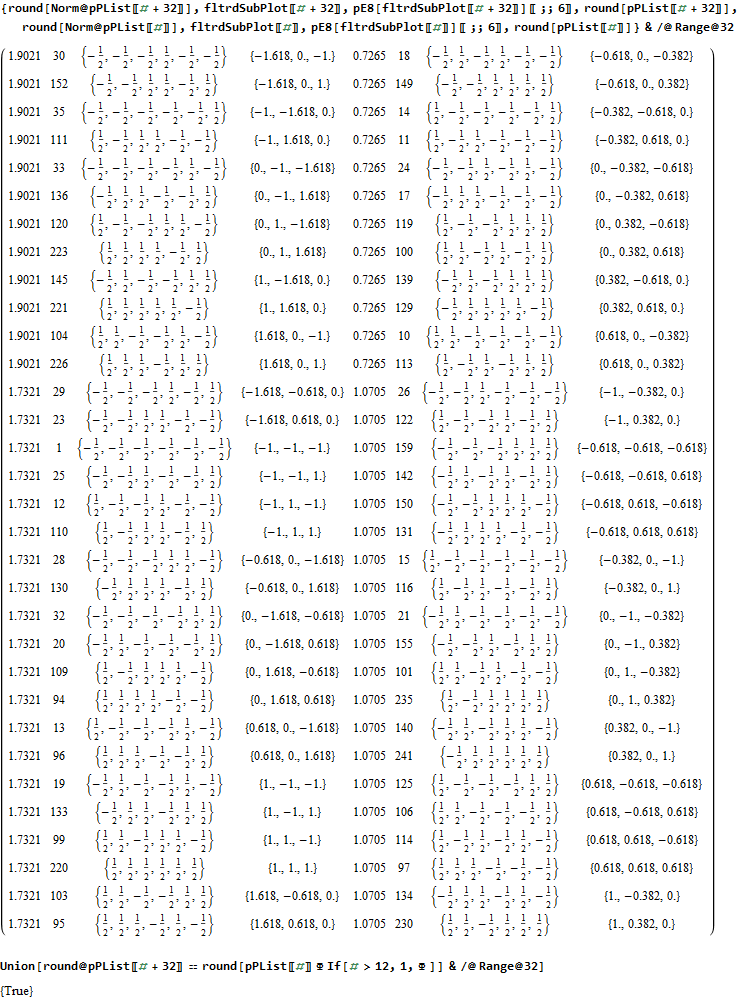The last line of the data set above shows that the outer data (left) is the same as the inner data set multiplied by a factor of Φ when the first 12 (shorter 3D Norm’d)  inner vertices (right) are multiplied by an additional factor of Φ.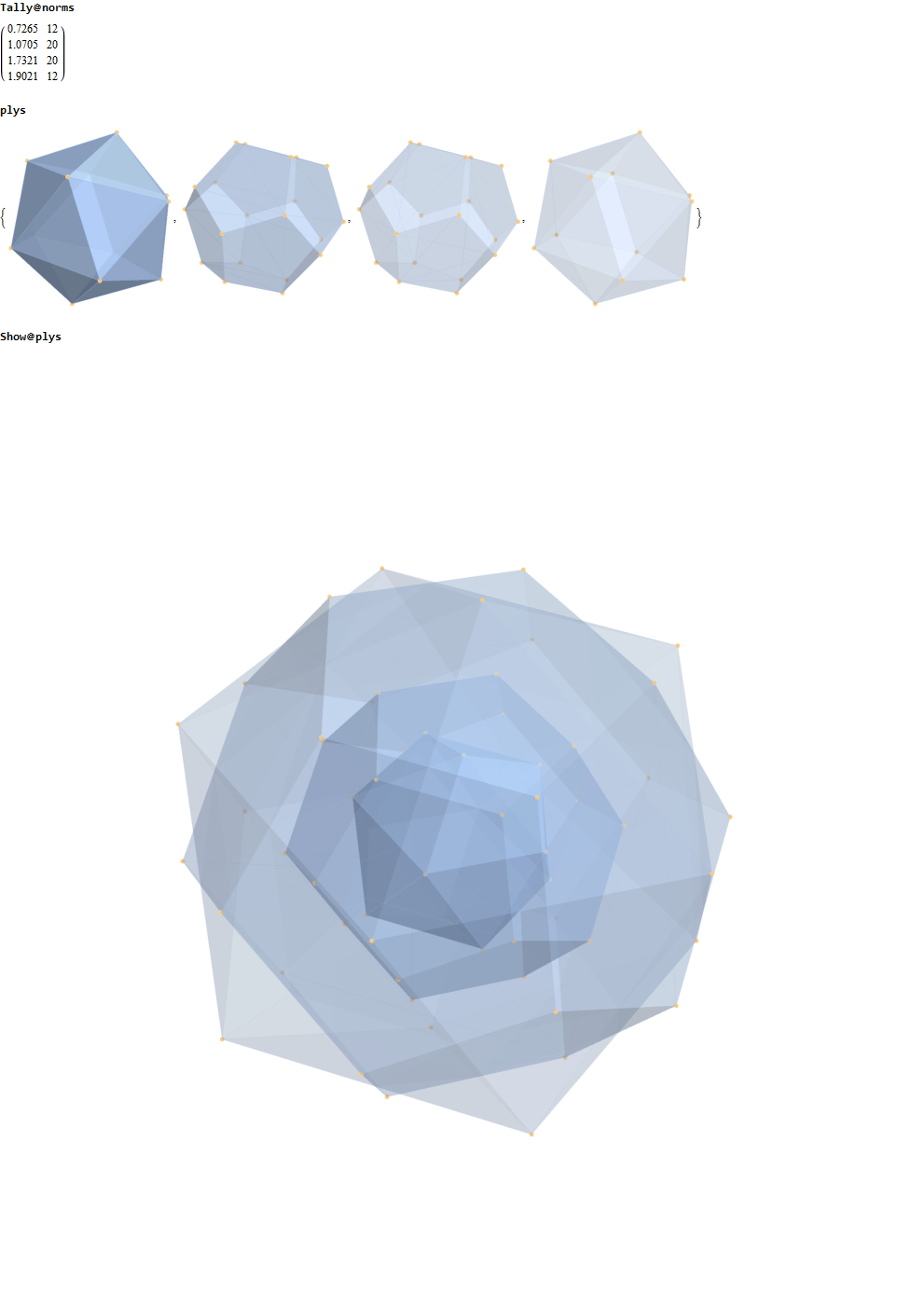#### This explains the lack of isomorphism, but why is it not like the D6 to H3+H3Φ and E8 to H4+H4Φ in the symmetry of Φ scaling?

The best explanation I can give involves the pattern of left vs. right 3 digits in the 6-Cube E8 vertices. This is due to the left right symmetry of the basis vectors applied to binary pattern of half integer vertices in the 6-Cube.

All vertices have a consistent pattern that differentiates the 20 longer Norm’d vertices from the 12 shorter ones. Specifically, the shorter 12 that have the inner ones needing the added factor of Φ always show that:

• the right inner vertices all have an even number in 6 +/- elements but not always evenly split across the left and right
• the left outer vertices all have an odd number of in 6 +/- elements  always unevenly split across the left and right

Whereas, the longer 20 that match the Φ scaling reverse the pattern where:

• the right inner vertices all have an odd number in 6 of +/- elements  always unevenly split across the left and right
• the left outer vertices all have an even number in 6 of +/- elements but not always evenly split across the left and right

Another related fact in the folding pattern of the 6-Cube emerges when we note that since E8 contains 128 1/2 integer BC8 (the 8 Demi-Cube). In addition, we note that the 6D complement of BC8 and the 6-Cube is the same set of vertices. This means we get the same projection using all 128 vertices of the 6D trimmed BC8 as we do with the 64 of the 6-Cube, except now there are 768 6D Norm’d unit length edges rather than 192. Interestingly, that means that similar to E8 containing simultaneous copies of 4 600-Cells (a left and right pair scaled at Φ), E8 contains 4 simultaneous copies of the rhombic triacontahedron after adding the Φ scaling on the two sets of 12 inner BC8 vertices).

#### Wow – that is nice! Remember, you heard it here first!

If we try this idea with the complement of the integer elements of E8, namely the 112  6D trimmed D8 vertices complemented with 60 D6 vertices, the 6D trimmed 52 vertex complement contains 4 identical copies of the excluded 16 generator vertices of E8 {+/-1,0,0,0,0,0,0,0} permutations (plus 4 {0,0,0,0,0,0,0,0} vertices).

As you can see, the folding of the integer elements of E8 do not fold the same as the 1/2 integer elements, and in retrospect, we probably should not expect them to.

# Fun with Tutte-Coxeter, Beordijk-Coxeter, E8 and H4

In reference to a G+ post by Baez (w/Greg Egan), it’s interesting to note the link to E8’s outer ring of the Petrie projection of a split real even E8, which creates a Beordijk-Coxeter helix.

Beordijk-Coxeter helix in 2D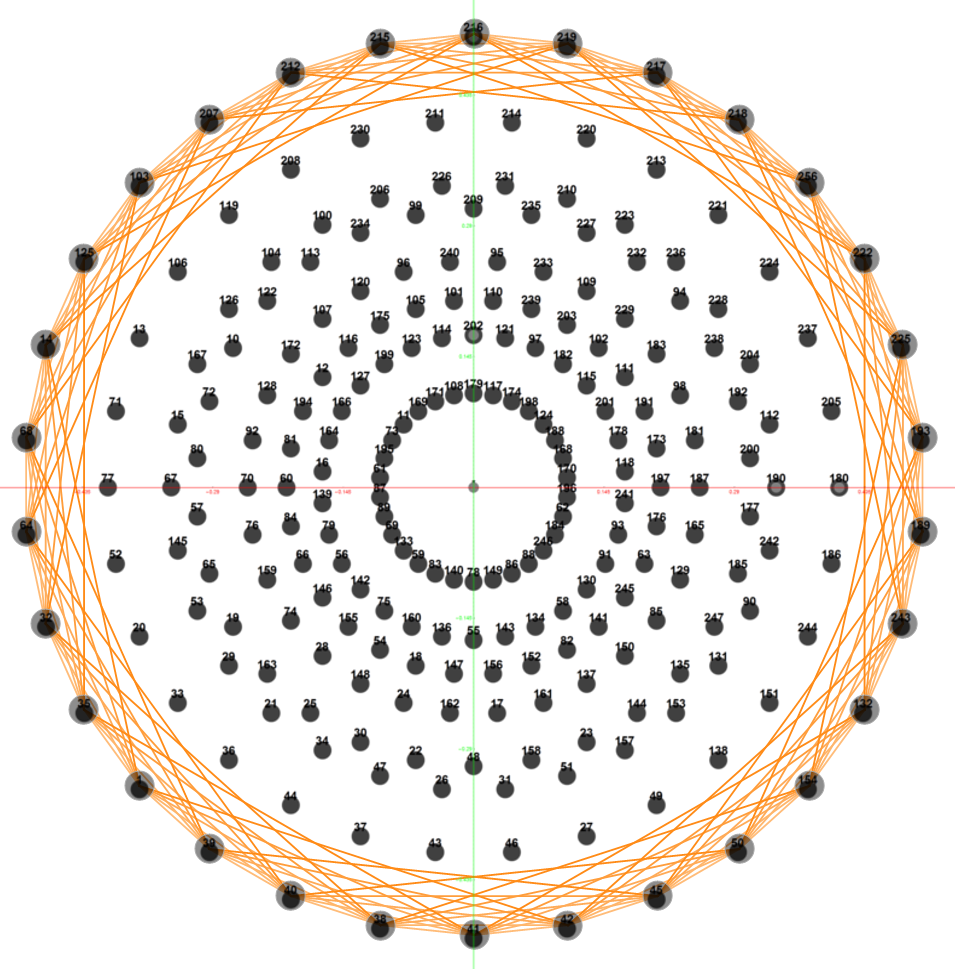Beordijk-Coxeter helix in 3DThe Beordijk-Coxeter helix connects the nearest 6 vertices on the outer ring. The Tutte-Coxeter graph is created in 3 (blk,grn,red) sets of edges by taking the (outer) ring and skipping (6,8,12) or counting (7,9,13) vertices. It shows there are 2 perfect pentagons and 1 pentagram (with different radii due to the difference in distance between the sets of vertices used).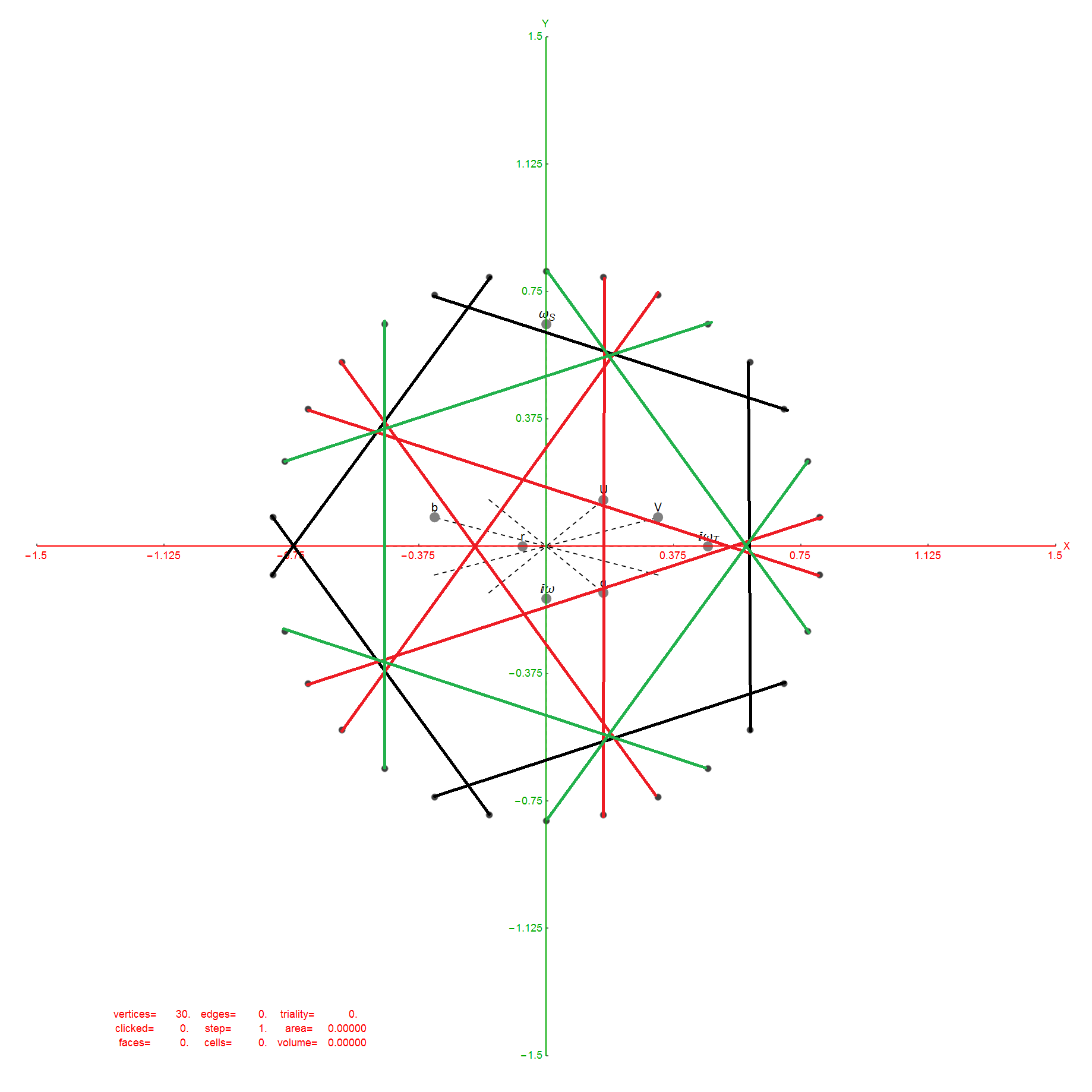Of course, the crystallographic E8 is manifestly related to the 5 fold symmetry of the pentagon, with its integral relationship to the non-crystallographic H4 group (and its Coxeter-Dynkin diagram) through E8 to H4 folding using the Golden ratio Phi.

It is interesting to note that the skipping of 5+(1,3,7) vertices is similar to the creation of the 120 (240) vertex positions of H4 (E8) Petrie projection by adding to the 24 vertices of the 8-cell and 16-cell (which make up the self-dual 24-cell) the 96 vertices of the Snub 24-cell. This is done through 4 rotations skipping 5 vertices.

Also notice the (1,3,7) are the number of the imaginary parts of Complex, Quaternion, and Octonion numbers, also integrally related to E8.